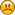Hello Guest

Model rendering from +z to -z and rotating around a point doesnt showing.

• 0 Replies
• 337 ViewsJoshi Elijah

•• 7Model rendering from +z to -z and rotating around a point doesnt showing.
« on: December 04, 2018, 06:21:55 »
Hi,

I am trying to rendering a model but it is projecting behind the camera, i am thinking of the problem being in the projection matrix from the link having the same problem https://stackoverflow.com/questions/23187262/lwjgl-projection-matrix-nothing-happens. I modified the projection matrix but still nothing happens, this is my projection matrix
Code: [Select]

private static final float FOV = 70;
private static final float NEAR_PLANE = 0.1f;
private static final float FAR_PLANE = 10000;

private void createProjectionMatrix() {
float aspectRatio = (float) Display.getWidth() / (float) Display.getHeight();
float y_scale = (float) ((1f / Math.tan(Math.toRadians(FOV / 2f))) * aspectRatio);
float x_scale = y_scale / aspectRatio;
float frustum_length = FAR_PLANE - NEAR_PLANE;

float bottom = -NEAR_PLANE * (float) Math.tan(Math.toRadians(FOV) / 2f);
float top = -bottom;
float left = aspectRatio * bottom;
float right = -left;

projectionMatrix = new Matrix4f();
projectionMatrix.m00 = x_scale;
projectionMatrix.m11 = y_scale;
projectionMatrix.m20 = (right+left) / (right-left); //
projectionMatrix.m21 = (top+bottom) / (top-bottom); //
projectionMatrix.m22 = -((FAR_PLANE + NEAR_PLANE) / frustum_length);
projectionMatrix.m23 = -1;
projectionMatrix.m32 = -((2 * NEAR_PLANE * FAR_PLANE) / frustum_length);
projectionMatrix.m33 = 0;
}

Can someone help me ?this is important for me.